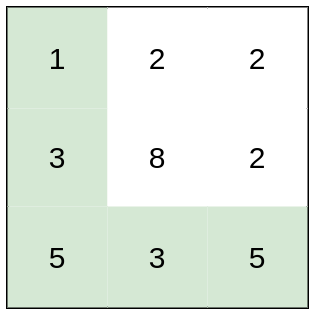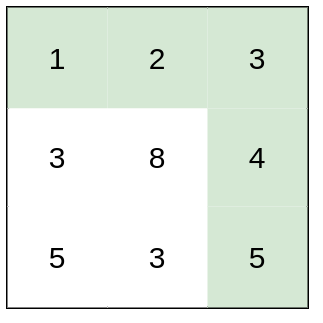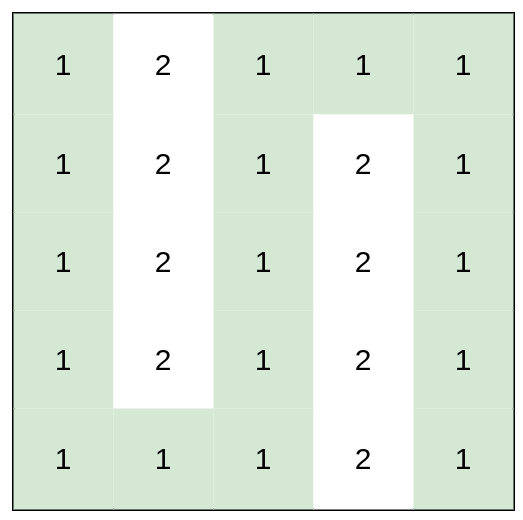## 1631. Path With Minimum Effort

You are a hiker preparing for an upcoming hike. You are given `heights`, a 2D array of size `rows x columns`, where `heights[row][col]` represents the height of cell `(row, col)`. You are situated in the top-left cell, `(0, 0)`, and you hope to travel to the bottom-right cell, `(rows-1, columns-1)` (i.e., 0-indexed). You can move up, down, left, or right, and you wish to find a route that requires the minimum effort.

A route's effort is the maximum absolute difference in heights between two consecutive cells of the route.

Return the minimum effort required to travel from the top-left cell to the bottom-right cell.

Example 1:```Input: heights = [[1,2,2],[3,8,2],[5,3,5]]
Output: 2
Explanation: The route of [1,3,5,3,5] has a maximum absolute difference of 2 in consecutive cells.
This is better than the route of [1,2,2,2,5], where the maximum absolute difference is 3.
```

Example 2:```Input: heights = [[1,2,3],[3,8,4],[5,3,5]]
Output: 1
Explanation: The route of [1,2,3,4,5] has a maximum absolute difference of 1 in consecutive cells, which is better than route [1,3,5,3,5].
```

Example 3:```Input: heights = [[1,2,1,1,1],[1,2,1,2,1],[1,2,1,2,1],[1,2,1,2,1],[1,1,1,2,1]]
Output: 0
Explanation: This route does not require any effort.
```

Constraints:

• `rows == heights.length`
• `columns == heights[i].length`
• `1 <= rows, columns <= 100`
• `1 <= heights[i][j] <= 106`

## Rust Solution

``````struct Solution;

use std::cmp::Reverse;
use std::collections::BinaryHeap;

impl Solution {
fn minimum_effort_path(heights: Vec<Vec<i32>>) -> i32 {
let n = heights.len();
let m = heights.len();
let mut visited: Vec<Vec<bool>> = vec![vec![false; m]; n];
let mut queue: BinaryHeap<(Reverse<i32>, usize, usize)> = BinaryHeap::new();
let mut res = 0;
queue.push((Reverse(0), 0, 0));
while let Some((Reverse(effort), i, j)) = queue.pop() {
res = res.max(effort);
if i == n - 1 && j == m - 1 {
break;
}
visited[i][j] = true;
if i > 0 && !visited[i - 1][j] {
let diff = heights[i][j] - heights[i - 1][j];
queue.push((Reverse(diff.abs()), i - 1, j));
}
if j > 0 && !visited[i][j - 1] {
let diff = heights[i][j] - heights[i][j - 1];
queue.push((Reverse(diff.abs()), i, j - 1));
}
if i + 1 < n && !visited[i + 1][j] {
let diff = heights[i][j] - heights[i + 1][j];
queue.push((Reverse(diff.abs()), i + 1, j));
}
if j + 1 < m && !visited[i][j + 1] {
let diff = heights[i][j] - heights[i][j + 1];
queue.push((Reverse(diff.abs()), i, j + 1));
}
}
res
}
}

#[test]
fn test() {
let heights = vec_vec_i32![[1, 2, 2], [3, 8, 2], [5, 3, 5]];
let res = 2;
assert_eq!(Solution::minimum_effort_path(heights), res);
let heights = vec_vec_i32![[1, 2, 3], [3, 8, 4], [5, 3, 5]];
let res = 1;
assert_eq!(Solution::minimum_effort_path(heights), res);
let heights = vec_vec_i32![
[1, 2, 1, 1, 1],
[1, 2, 1, 2, 1],
[1, 2, 1, 2, 1],
[1, 2, 1, 2, 1],
[1, 1, 1, 2, 1]
];
let res = 0;
assert_eq!(Solution::minimum_effort_path(heights), res);
}
``````

Having problems with this solution? Click here to submit an issue on github.